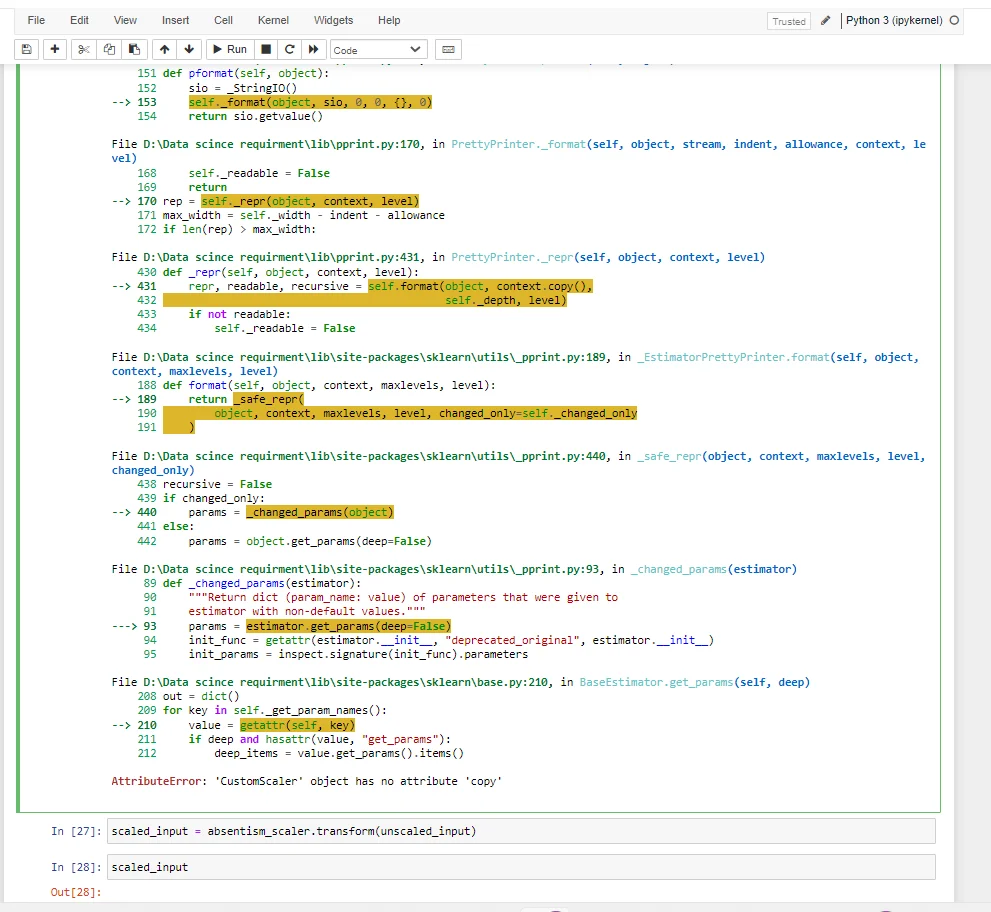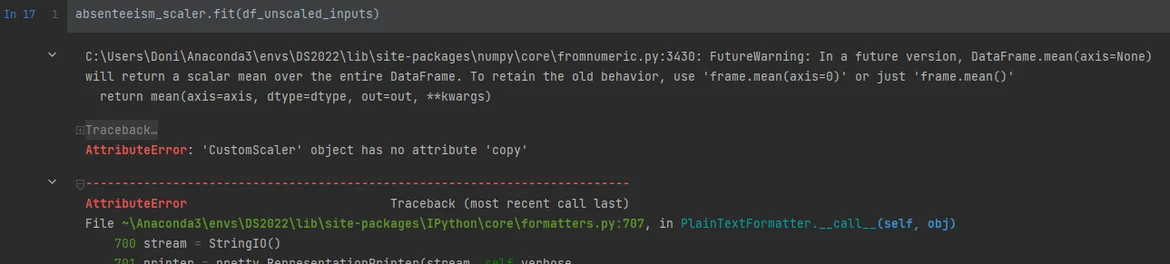20 Nov 2022

Posted on:

07 Nov 2022

0

# Resolved:creating custom CustomScalar

when i excute thi line code  absentism_scaler.fit(unscaled_input)
it give me error

``````AttributeError: 'CustomScaler' object has no attribute 'copy'
``````Instructor
Posted on:

10 Nov 2022

0

Hi Mostafa!
Thanks for reaching out.

This error stems from the code you've executed previously in your *.ipynb file. We are aware that this may mean that you have made one of potentially a number of errors, but it is not exactly so. From the screenshot you've shared, it seems that you may have not created the unscaled_inputs in the way shown in the course. Therefore, can you please revise/restart the kernel and re-run all cells in the suggested order, to ensure that this variable contains the relevant content?

Tsvetelin

Posted on:

20 Nov 2022

0

I have encounter same issue.

i have followed exactly the same, and also _ _ init _ _ using

``````self.scaler = StandardScaler(copy=copy, with_mean=with_mean, with_std=with_std)
``````

maybe due to this FutureWarning?Posted on:

20 Nov 2022

3

try this
-----------------------------------------------------------------------------------------------------
class CustomScaler(BaseEstimator, TransformerMixin):

def __init__(self, columns, copy=True, with_mean=True, with_std=True):
self.columns = columns
self.copy = copy
self.with_mean = with_mean
self.with_std = with_std

def fit(self, X, y=None):
self.scaler = StandardScaler(copy= self.copy, with_mean=self.with_mean, with_std=self.with_std)
self.scaler.fit(X[self.columns], y)
self.mean_ = np.mean(X[self.columns])
self.var_ = np.var(X[self.columns])
return self

def transform(self, X, y=None, copy=None):
init_col_order = X.columns
X_scaled = pd.DataFrame(self.scaler.transform(X[self.columns]), columns=self.columns)
X_not_scaled = X.loc[:, ~X.columns.isin(self.columns)]
return pd.concat([X_not_scaled, X_scaled], axis=1)[init_col_order]
-----------------------------------------------------------------------------------------------
i modified the code from the video using this solution on stack overflow, and it worked.
https://stackoverflow.com/questions/70587280/sklearn-attributeerror-customscaler-object-has-no-attribute-copy